## CSTR

Original source of the model: A. Uppal, W.H. Ray, A.B. Poore: On the dynamic behavior of continuous stirred tank reactors. Chem. Eng. Sci. 29 (1974) 967-985.

A branching diagram is shown in Figure 1. We see a branch of stationary solutions with two Hopf bifurcation points, for which the parameter values and initial periods are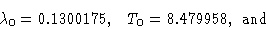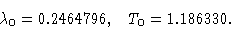The two Hopf points are connected by a branch of periodic orbits (indicated by two parts in Figure 1). This example exhibits both subcritical and supercritical bifurcation. At the right Hopf point there is a soft generation of limit cycles (for decreasing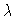), whereas at the left Hopf point the loss of stability is hard. The branch of stationary solutions has two turning points close to each other with a difference inof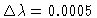. As is hardly seen in Figure 1, the lower turning point is above the left Hopf point. The hysteresis part of the branching diagram thus lies fully in the unstable range between the Hopf points.

In Figures 2 and 3, simulations are performed with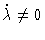. The soft loss of stability in Figure 3 looks like a hard loss. The relatively steep transition is due to a slow reaction of the system. Note that the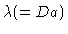scaling that corresponds to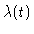in Figure 2 is the same as in Figure 1. The phase condition of the calculations in Figure 1 is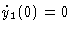, here fixing the maximum of y1(t). Hence there is an immediate correspondence between Figure 1 and Figure 2 in size, scaling, and dynamical behavior. This is similar in Figure 3, except for the reverse time; flip Figure 3 and it matches Figure 1 and 2.

Continuous" refers to a continuous flow entering (and leaving) the reactor--that is, a CSTR is an open system. Human beings and other living organisms that have input of reactants (nutrients) and output of products (wastes) are complex examples of CSTRs. The exponential term in the equations reflects an infinite activation energy.

Figure 1
Branching diagram y1(0) versus Da. One branch of stationary solutions, and two branches of periodic orbits branching off at two Hopf bifurcations.

Figure 2
y1(t) simulation for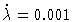,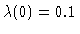,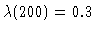with initial values y1(0)=0.1644, y2(0)=0.6658

Figure 3
y1(t) simulation for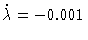,,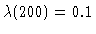with initial values y1(0)=0.9279, y2(0)=3.76

This Example
Figure 1
Figure 2
Figure 3